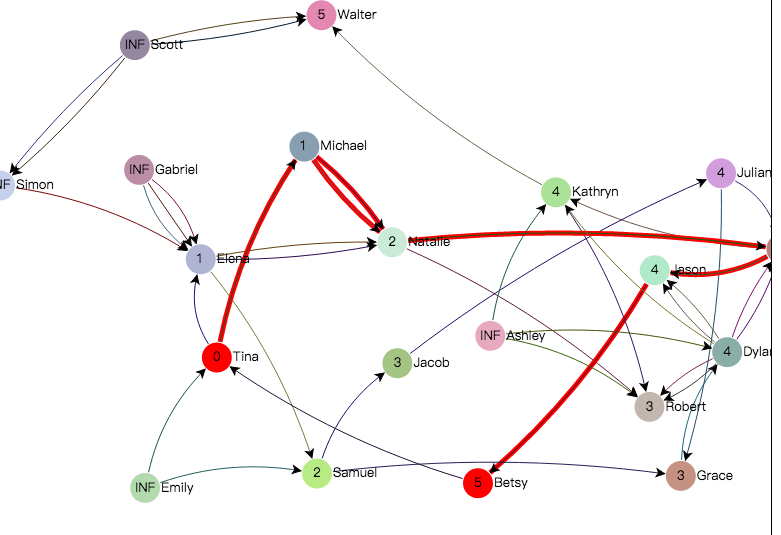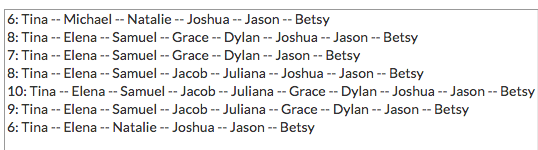# gojs实现最短路径寻址实例

2017/03/28 18:52

## JS

  function init() {
if (window.goSamples) goSamples();  // init for these samples -- you don't need to call this
var $= go.GraphObject.make; // for conciseness in defining templates myDiagram =$(go.Diagram, "myDiagramDiv", // must be the ID or reference to div
{
initialAutoScale: go.Diagram.UniformToFill,
contentAlignment: go.Spot.Center,
layout: $(go.ForceDirectedLayout, { defaultSpringLength: 10 }), maxSelectionCount: 2 }); // define the Node template myDiagram.nodeTemplate =$(go.Node, "Horizontal",
{ locationSpot: go.Spot.Center,  // Node.location is the center of the Shape
locationObjectName: "SHAPE",
selectionChanged: nodeSelectionChanged },
$(go.Panel, "Auto",$(go.Shape, "Ellipse",
{ name: "SHAPE",
fill: "lightgray",  // default value, but also data-bound
stroke: "transparent",  // modified by highlighting
strokeWidth: 2,
desiredSize: new go.Size(30, 30),
portId: "" },  // so links will go to the shape, not the whole node
new go.Binding("fill", "isSelected", function(s, obj) { return s ? "red" : obj.part.data.color; }).ofObject()),
$(go.TextBlock, new go.Binding("text", "distance", function(d) { if (d === Infinity) return "INF"; else return d | 0; }))),$(go.TextBlock,
new go.Binding("text")));

$(go.Link, { selectable: false, // links cannot be selected by the user curve: go.Link.Bezier, layerName: "Background" // don't cross in front of any nodes },$(go.Shape,  // this shape only shows when it isHighlighted
{ isPanelMain: true, stroke: null, strokeWidth: 5 },
new go.Binding("stroke", "isHighlighted", function(h) { return h ? "red" : null; }).ofObject()),
$(go.Shape, // mark each Shape to get the link geometry with isPanelMain: true { isPanelMain: true, stroke: "black", strokeWidth: 1 }, new go.Binding("stroke", "color")),$(go.Shape, { toArrow: "Standard" })
);

// Override the clickSelectingTool's standardMouseSelect
// If less than 2 nodes are selected, always add to the selection
myDiagram.toolManager.clickSelectingTool.standardMouseSelect = function() {
var diagram = this.diagram;
if (diagram === null || !diagram.allowSelect) return;
var e = diagram.lastInput;
var count = diagram.selection.count;
var curobj = diagram.findPartAt(e.documentPoint, false);
if (curobj !== null) {
if (count < 2) {  // add the part to the selection
if (!curobj.isSelected) {
var part = curobj;
if (part !== null) part.isSelected = true;
}
} else {
if (!curobj.isSelected) {
var part = curobj;
if (part !== null) diagram.select(part);
}
}
} else if (e.left && !(e.control || e.meta) && !e.shift) {
// left click on background with no modifier: clear selection
diagram.clearSelection();
}
}

generateGraph();

// select two nodes that connect from the first one to the second one
var num = myDiagram.model.nodeDataArray.length;
var node1 = null;
var node2 = null;
for (var i = 0; i < num; i++) {
node1 = myDiagram.findNodeForKey(i);
var distances = findDistances(node1);
for (var j = 0; j < num; j++) {
node2 = myDiagram.findNodeForKey(j);
var dist = distances.getValue(node2);
if (dist > 1 && dist < Infinity) {
node1.isSelected = true;
node2.isSelected = true;
break;
}
}
if (myDiagram.selection.count > 0) break;
}
}

function generateGraph() {
var names = [
"Joshua", "Kathryn", "Robert", "Jason", "Scott", "Betsy", "John",
"Walter", "Gabriel", "Simon", "Emily", "Tina", "Elena", "Samuel",
"Jacob", "Michael", "Juliana", "Natalie", "Grace", "Ashley", "Dylan"
];

var nodeDataArray = [];
for (var i = 0; i < names.length; i++) {
nodeDataArray.push({ key: i, text: names[i], color: go.Brush.randomColor(128, 240) });
}

var num = nodeDataArray.length;
for (var i = 0; i < num * 2; i++) {
var a = Math.floor(Math.random() * num);
var b = Math.floor(Math.random() * num / 4) + 1;
linkDataArray.push({ from: a, to: (a + b) % num, color: go.Brush.randomColor(0, 127) });
}

}

// There are three bits of functionality here:
// 1: findDistances(Node) computes the distance of each Node from the given Node.
//    This function is used by showDistances to update the model data.
// 2: findShortestPath(Node, Node) finds a shortest path from one Node to another.
//    This uses findDistances.  This is used by highlightShortestPath.
// 3: collectAllPaths(Node, Node) produces a collection of all paths from one Node to another.
//    This is used by listAllPaths.  The result is remembered in a global variable
//    which is used by highlightSelectedPath.  This does not depend on findDistances.

// Returns a Map of Nodes with distance values from the given source Node.
// Assumes all links are unidirectional.
function findDistances(source) {
var diagram = source.diagram;
// keep track of distances from the source node
var distances = new go.Map(go.Node, "number");
var nit = diagram.nodes;
while (nit.next()) {
var n = nit.value;
}
// the source node starts with distance 0
// keep track of nodes for which we have set a non-Infinity distance,
// but which we have not yet finished examining
var seen = new go.Set(go.Node);

// keep track of nodes we have finished examining;
// this avoids unnecessary traversals and helps keep the SEEN collection small
var finished = new go.Set(go.Node);
while (seen.count > 0) {
// look at the unfinished node with the shortest distance so far
var least = leastNode(seen, distances);
var leastdist = distances.getValue(least);
// by the end of this loop we will have finished examining this LEAST node
seen.remove(least);
// look at all Links connected with this node
while (it.next()) {
// skip nodes that we have finished
if (finished.contains(neighbor)) continue;
var neighbordist = distances.getValue(neighbor);
// assume "distance" along a link is unitary, but could be any non-negative number.
var dist = leastdist + 1;  //Math.sqrt(least.location.distanceSquaredPoint(neighbor.location));
if (dist < neighbordist) {
// if haven't seen that node before, add it to the SEEN collection
if (neighbordist === Infinity) {
}
// record the new best distance so far to that node
}
}
}

return distances;
}

// This helper function finds a Node in the given collection that has the smallest distance.
function leastNode(coll, distances) {
var bestdist = Infinity;
var bestnode = null;
var it = coll.iterator;
while (it.next()) {
var n = it.value;
var dist = distances.getValue(n);
if (dist < bestdist) {
bestdist = dist;
bestnode = n;
}
}
return bestnode;
}

// Find a path that is shortest from the BEGIN node to the END node.
// (There might be more than one, and there might be none.)
function findShortestPath(begin, end) {
// compute and remember the distance of each node from the BEGIN node
distances = findDistances(begin);

// now find a path from END to BEGIN, always choosing the adjacent Node with the lowest distance
var path = new go.List();
while (end !== null) {
var next = leastNode(end.findNodesInto(), distances);
if (next !== null) {
if (distances.getValue(next) < distances.getValue(end)) {
path.add(next);  // making progress towards the beginning
} else {
next = null;  // nothing better found -- stop looking
}
}
end = next;
}
// reverse the list to start at the node closest to BEGIN that is on the path to END
// NOTE: if there's no path from BEGIN to END, the first node won't be BEGIN!
path.reverse();
return path;
}

// Recursively walk the graph starting from the BEGIN node;
// when reaching the END node remember the list of nodes along the current path.
// Finally return the collection of paths, which may be empty.
// This assumes all links are unidirectional.
function collectAllPaths(begin, end) {
var stack = new go.List(go.Node);
var coll = new go.List(go.List);

function find(source, end) {
source.findNodesOutOf().each(function(n) {
if (n === source) return;  // ignore reflexive links
if (n === end) {  // success
var path = stack.copy();
path.add(end);  // finish the path at the end node
coll.add(path);  // remember the whole path
} else if (!stack.contains(n)) {  // inefficient way to check having visited
stack.add(n);  // remember that we've been here for this path (but not forever)
find(n, end);
stack.removeAt(stack.count - 1);
}  // else might be a cycle
});
}

stack.add(begin);  // start the path at the begin node
find(begin, end);
return coll;
}

// Return a string representation of a path for humans to read.
function pathToString(path) {
var s = path.length + ": ";
for (var i = 0; i < path.length; i++) {
if (i > 0) s += " -- ";
s += path.elt(i).data.text;
}
return s;
}

// When a node is selected show distances from the first selected node.
// When a second node is selected, highlight the shortest path between two selected nodes.
// If a node is deselected, clear all highlights.
function nodeSelectionChanged(node) {
var diagram = node.diagram;
if (diagram === null) return;
diagram.clearHighlighteds();
if (node.isSelected) {
// when there is a selection made, always clear out the list of all paths
var sel = document.getElementById("myPaths");
sel.innerHTML = "";

// show the distance for each node from the selected node
var begin = diagram.selection.first();
showDistances(begin);

if (diagram.selection.count === 2) {
var end = node;  // just became selected

// highlight the shortest path
highlightShortestPath(begin, end);

// list all paths
listAllPaths(begin, end);
}
}
}

// Have each node show how far it is from the BEGIN node.
function showDistances(begin) {
// compute and remember the distance of each node from the BEGIN node
distances = findDistances(begin);

// show the distance on each node
var it = distances.iterator;
while (it.next()) {
var n = it.key;
var dist = it.value;
myDiagram.model.setDataProperty(n.data, "distance", dist);
}
}

// Highlight links along one of the shortest paths between the BEGIN and the END nodes.
function highlightShortestPath(begin, end) {
highlightPath(findShortestPath(begin, end));
}

// List all paths from BEGIN to END
function listAllPaths(begin, end) {
// compute and remember all paths from BEGIN to END: Lists of Nodes
paths = collectAllPaths(begin, end);

// update the Selection element with a bunch of Option elements, one per path
var sel = document.getElementById("myPaths");
sel.innerHTML = "";  // clear out any old Option elements
paths.each(function(p) {
var opt = document.createElement("option");
opt.text = pathToString(p);
});
sel.onchange = highlightSelectedPath;
}

// A collection of all of the paths between a pair of nodes, a List of Lists of Nodes
var paths = null;
// This is only used for listing all paths for the selection onchange event.

// When the selected item changes in the Selection element,
// highlight the corresponding path of nodes.
function highlightSelectedPath() {
var sel = document.getElementById("myPaths");
var idx = sel.selectedIndex;
var opt = sel.options[idx];
var val = opt.value;
highlightPath(paths.elt(sel.selectedIndex));
}

// Highlight a particular path, a List of Nodes.
function highlightPath(path) {
myDiagram.clearHighlighteds();
for (var i = 0; i < path.count - 1; i++) {
var f = path.elt(i);
var t = path.elt(i + 1);
f.findLinksTo(t).each(function(l) { l.isHighlighted = true; });
}
}

## HTML

<div id="sample">
<div id="myDiagramDiv" style="border: solid 1px black; background: white; width: 100%; height: 700px"></div>
Click on a node to show distances from that node to each other node.
Click on a second node to show a shortest path from the first node to the second node.
(Note that there might not be any path between the nodes.)
<p>
Clicking on a third node will de-select the first two.
</p><p>
Here is a list of all paths between the first and second selected nodes.
Select a path to highlight it in the diagram.
</p>
<select id="myPaths" style="min-width:100px" size="10"></select>
</div>

## 效果### 作者的其它热门文章

0
0 收藏

0 评论
0 收藏
0